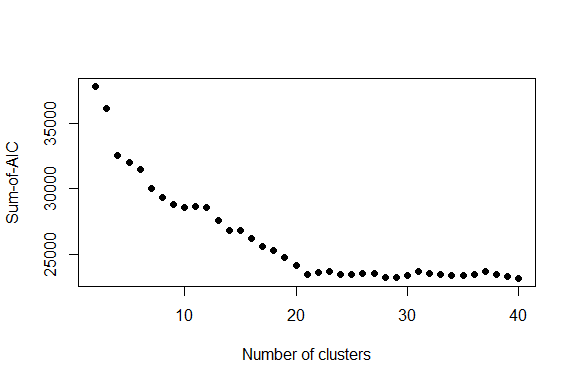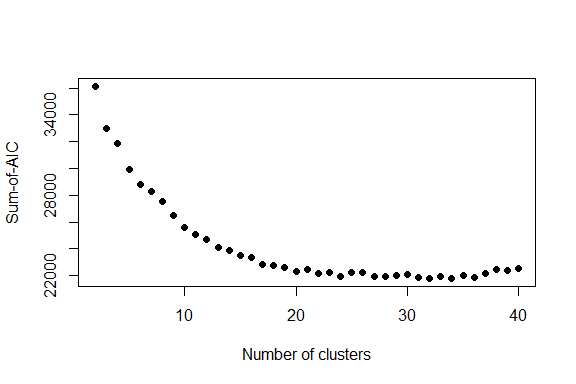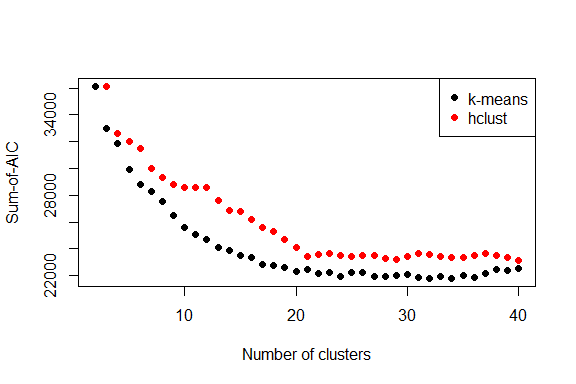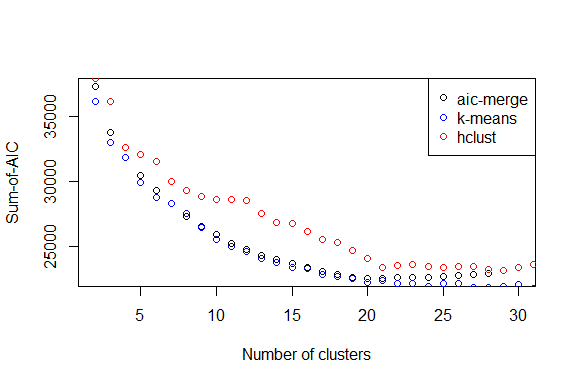Optimus workflow

2017-03-23

This vignette outlines a typical workflow that one might use optimus for. Using an ecological example, it shows how optimus can be used for assessment and diagnostics of competing clustering solutions. The workflow includes:

• find an optimal partitioning solution amoung competing solutions
• identify characteristic species
• refine a classification by merging clusters that increase predictive performance

Optimus is built on the premise that a good clustering solution (i.e. a classification) should provide information about the composition and abundance of the multivariate data it is classifying. A natural way to formalize this is with a predictive model, where group membership (clusters) is the predictor, and the multivariate data (site by variables matrix) is the response. optimus uses a multivariate glm framework to fit predictive models, the performance of which inform the classificaiton assessment and diagnostic outputs. The sections below provide further information on the individual componetns of the workflow. Lyons et al. (2016) provides theoretical background, a detailed description of the methodology, and application of the methods on both real and simulated ecological multivariate abundance data.

Supported data types

At present, optimus supports the following data types/error distributions:

• Gaussian (‘normal’ data)
• Negatvie binomial & Poisson (count data)
• Binomial (binary, presense/absence and trials data)
• Ordinal (ordinal, cover-abundance and categorical data)

Note that while there is an ecologcial focus to these data exmaples, in a more general sense, this package handle any set of data for i observations of j variables of the above data types. Make sure optimus is loaded at this point.

library(optimus)

Finding an optimal partitioning solution

Finding the ‘best’ clustering solution amoung a number of alternatives or competing solutions is a common problem. This problem is the underlying basis of optimus. The find_optimal() function takes one or more clustering solutions and calculates a ‘goodness of fit’ metric for them called sum-of-AIC. Sum-of-AIC is motivated as an estimate of Kullback-Leibler distance, so we posit that the clustering solution that minimises the sum-of-AIC value is the best - simple, but objective and repeatable. Check out ?find_optimal to get a feel for the required inputs and expected outputs. The underlying premise is that the clustering solutions are used to predict the multivariate data, so that data is therefore required as an input. We’ll use the ?swamps data that comes with optimus.

data(swamps)
swamps <- swamps[,-1] # get rid of the site ID

Now we need some clustering solutions to assess. find_optimal() can be supplied with clustering solutions in two different ways: 1) a single object on which the cutree() function can work (this is a common output class for many clustering funcitons in R); or 2) simply a list of clustering solutions. Let’s try the first method.

find_optimal() using cutree() method

We create the cutree-able object with the hclust() and dist() functions - this calculates a distance matrix on the abundance data, and then performs a heirarchical clustering routine.

swamps_hclust <- hclust(d = dist(x = log1p(swamps), method = "canberra"),
method = "complete")

Now, this is probably not a particularly smart way of clustering ecological data, but does work without installing any additional packages. You might like to check out the {vegan} and {cluster} packages - for example bray curtis distance (e.g. vegan::vegdist) and flexible-beta clustering (e.g. cluster::agnes) is a popular choice. Moving on, lets now calculate sum-of-AIC using the inbuilt cutree() functionaliy in find_optimal(). Through out optimus, you’ll notice that messeges print to confirm the types of procedures you’re doing, you can turn them off if you like, and I supress them in this vignette

swamps_hclust_aics <- find_optimal(data = swamps,
clustering = swamps_hclust,
family = "poisson",
cutreeLevels = 2:40)

We pass the original abundance data and the clustering object, and we also specify that a Poisson (negative binomial might be a better choice in reality) error distribution should be used and we want to test clustering solutions with 2 to 40 groups. We can view the results, plot manually from the resulting object, or use the inbuilt plotting function, which plots the results out to an east-to-interpret graph.

##    sum_aic nclusters
## 1 37827.58         2
## 2 36137.12         3
## 3 32577.78         4
## 4 32036.79         5
## 5 31491.49         6
## 6 30012.63         7
plot(swamps_hclust_aics)So we can see from this clustering effort that around 21 clusters is the best solution - after that the predictive performance of the clustering solution does not improve. Now let’s try again, but this time supply a list of clustering solutions.

find_optimal() by supplying a list

Most non-heirarchical clustering functions do not have a cutree() method. If you are passing a list of clustering solutions to find_optimal(), you must ensure that the list elements are a vector of cluster labels that matches the number of rows in the data. Let’s use k-means as an example.

swamps_kmeans <- lapply(X = 2:40,
FUN = function(x, data) {stats::kmeans(x = data, centers = x)$cluster}, data = swamps) This is just a little trick using lapply() that creates a k-means clustering solution for each of 2 to 40 groups, dumping them all into a list. For exmaple, look at the allocation for the 4-group solution table(swamps_kmeans[]) ## ## 1 2 3 4 ## 15 23 14 2 Now we calculate and plot sum-of-AIC in the same way as before, but pass the list instead. swamps_kmeans_aics <- find_optimal(data = swamps, clustering = swamps_kmeans, family = "poisson") plot(swamps_kmeans_aics)So, similarly to before, it looks like around 20 clusters is again around the optimal solution. It’s nice when that happens! Note that you can just supply a single clustering solution, and the sum-of-AIC will still be calculated. We can utilise the points() method to plot them together too - it works in the same way as plot() on aicsums objects except that it just plots points over the top of a previous plot, so is useful for comparing multiple sets of clustering solutions. plot(swamps_kmeans_aics) points(swamps_hclust_aics, col = "red", pch = 16) legend("topright", legend = c("k-means", "hclust"), col = c("black", "red"), pch = 16)So according to sum-of-AIC, the k-means solutions are all just a bit better. Note also the other possible arguments K= and cutreeOveride= should the need arise. Determining characteristic species Let’s say at this point we have chosen our best classification - say one with 20 clusters, based on the previous exercise. Or perhaps you already have a classification and you just want to calculate characteristic species. get_characteristic() uses the same underlying predictive model framework as find_optimal() except that now we use it to extract the important variables, that is, the ones that the clustering solution has good predictive performance for. In Ecology, this is the process of determining characteristic species, also referred to as diagnostic or indicator species. The heirarchical solution is used here so you should get the same results, but there is a small chance it might be a little different. Per-cluster characteristic species The first type of characteristic species we will look at is ‘per-cluster’. This means that we will extract a list of characteristic speices for each cluster. Briefly, charactersitic species are defined by the coefficient values that correspond to each level of the clustering solution (remembering that we are fitting models where the data are the responses and the clustering solution is the predictor). Using the data and clustering we have used above, let’s make a solution with 20 clusters and then run get_characteristic() - we need to pass the multivariate data too. swamps_20groups <- cutree(tree = swamps_hclust, k = 20) swamps_char <- get_characteristic(data = swamps, clustering = swamps_20groups, family = "poisson", type = "per.cluster") print(swamps_char$clusterSolution1)
##                     variables coef_value      daic    stderr
## 1        Schoenus.brevifolius  3.1060803 265.19679 0.1221694
## 2  Chorizandra.sphaerocephala  2.8716796 467.93666 0.1373606
## 3       Lepidosperma.limicola  2.6625878 635.59129 0.1524986
## 4             Empodisma.minus  2.6390573 270.99127 0.1543033
## 5          Banksia.ericifolia  2.3353749 341.15909 0.1796053
## 6         Lepidosperma.neesii  2.3025851 564.16069 0.1825742
## 7      Gleichenia.microphylla  2.2686835 706.19536 0.1856953
## 8           Leptocarpus.tenax  2.1202635 293.33741 0.2000000
## 9    Leptospermum.juniperinum  1.4663371 241.78674 0.2773501
## 10          Melaleuca.squamea  1.2992830  80.27894 0.3015113
## 11              Banksia.robur  1.2039728 257.43420 0.3162278
## 12          Hakea.teretifolia  1.0986123 136.69087 0.3333333
## 13         Bauera.microphylla  0.9808293 345.90824 0.3535534
## 14    Leptospermum.squarrosum  0.9808293 405.67603 0.3535534
## 15          Entolasia.stricta  0.8472979 232.03233 0.3779645
## 16          Baeckea.linifolia  0.6931472 290.25843 0.4082483
## 17       Petrophile.pulchella  0.6931472 135.47135 0.4082483
## 18    Xanthorrhoea.resinifera  0.6931472 275.34378 0.4082483
## 19           Xyris.operculata  0.6931472 505.73708 0.4082483
## 20       Dillwynia.floribunda  0.5108256 188.25065 0.4472136

get_characteristic(..., pre.cluster=T) returns a list where the elements contain a data frame of characteristic species for each cluster group. Above, we’ve printed out the characteristic species for cluster 1 (the name in the list inherrits from the names in swamps_20groups). The species list is sorted by the size of the coefficient, but it’s dangerous to only consider that, because a big coefficient can sometimes mean a big nothing. So by default the output also includes delta AIC values (eplxained further below) and standard errors. We loosely define that the larger the coefficient (with larger delta AIC values and smaller standard errors guiding significance), the more characteristic that variable (species) is.

Remember the coefficent values might be on a link scale (see Details in ?get_characteristic) and that size is not everything. For example, in the characteristic species list we printed out above - the coefficient for cluster 1 has a mean effect of ~22 (exp(3.1060803) because the Poisson GLM uses a log-link) on Schoenus brevifolius; cluster 1 only has a mean effect of ~10 on Gleichenia microphylla, but a much higher delta AIC. So you would have to consider whether you want to put more weight on how big the effect of each cluster is versus how significant it is. It’s a little subjective, but characteristic species analysis usually is - there are probably ecologcial considerations in this example that are important (e.g. S. brevifolius is a fairly widespread sedge, and G. microphylla is a fern with a fairly small range).

Global characteristic species

Sometimes we might be interested more generally in the species that are important for a classification, without needing attribution to a particular cluster. In context of model-based approaches, this is actually a more natural way of considering characteristic variables. In an ecological sense, this type of characteristic species might be analgous to ‘high fidelity’ or ‘faithful’ species. This time we just fit the null model and then calculate delta AIC for each species (high values mean more significance). The syntax is as before, except we just modify the type= argument.

swamps_20groups <- cutree(tree = swamps_hclust, k = 20)
swamps_char_glob <- get_characteristic(data = swamps,
clustering = swamps_20groups,
family = "poisson",
type = "global")
##                        variables     dAIC
## 1              Ptilothrix.deusta 811.5695
## 2         Gleichenia.microphylla 706.1954
## 3          Lepidosperma.limicola 635.5913
## 4            Lepidosperma.neesii 564.1607
## 5             Baumea.teretifolia 546.1552
## 6               Xyris.operculata 505.7371
## 7     Chorizandra.sphaerocephala 467.9367
## 8             Lepyrodia.scariosa 442.8302
## 9           Baloskion.fastigiata 432.3520
## 10             Baeckea.imbricata 420.3051
## 11           Epacris.obtusifolia 414.8919
## 12       Leptospermum.squarrosum 405.6760
## 13 Gymnoschoenus.sphaerocephalus 383.7726
## 14             Lindsaea.linearis 368.5211
## 15            Bauera.microphylla 345.9082
## 16            Banksia.ericifolia 341.1591
## 17             Cassytha.glabella 321.6604
## 18             Almaleea.paludosa 303.3420
## 19             Leptocarpus.tenax 293.3374
## 20             Baeckea.linifolia 290.2584

As a side note, another appraoch would be to use a resampling approach to calculate bootstrapped p-values (e.g. using mvabund::manyglm()) the the significance levels are a bit more exact, but in general delta AIC performs pretty well.

Refining a classificaiton

The last part of this workflow is using the sum-of-AIC framework to refine a classificaiton - that is, to merge clusters such that the predictive performance of the model is improved. merge_clusters() will take a clustering solution as a starting point, and then generate all possible pairwise combinations of clusters, fit the models, and then merge the pair with the lowest delta sum-of-AIC value (i.e. the one that least improves the predicitve performance of the classification with respect to the data). So again we need to supply the data, a starting clustering solution, and optionally how many times we want to perform a merge. See ?merge_clusters for the defaults. So, going back to our heirarchical clustering solution, let’s start with a higher number of clusters and merge downwards from there.

swamps_30groups <- cutree(tree = swamps_hclust, k = 30)

swamps_aicmerge <- merge_clusters(data = swamps,
clustering = swamps_30groups,
family = "poisson",
n.iter = 29)

merge_clusters() returns a list, where each component is the clustering solution after each iteration (but the first component is the original solution). It will also tell you which clusters were merged in each iteration, but that’s supressed here. It can be slow if there are many clusters, but it gets faster and faster as the numebr of clusters get’s smaller. In this case we merged from 30 clusters down to 2 (29 iterations) to match our previous analyses. New clusters (merges) are given a new cluster label, from {9000, 9001, … n}. For example lets look at the cluster assignments after after 20 iterations.

table(swamps_aicmerge[])
##
##   25 9009 9013 9014 9015 9016 9017 9018 9019 9020
##    3    2    4    6    8    6    6    3    9    7

There’s only one of the original clusters left. Since the object is a list of clustering solutions, we can plug that straight into find_optimal(). Let’s look at how the AIC based merging compares to merging based on the classificaiotn heirarchy (remembering we calculated the sum-of-AICs back in the first section).

swamps_aicmerge_aics <- find_optimal(data = swamps,
clustering = swamps_aicmerge,
family = "poisson")

plot(swamps_aicmerge_aics, pch = 1)
points(swamps_hclust_aics, col = "red", pch = 1)
points(swamps_kmeans_aics, col = "blue", pch = 1)
legend("topright",
legend = c("aic-merge", "k-means", "hclust"),
col = c("black", "blue", "red"), pch = 1)Note the use of the generic points() function again. Taking it further, you might repeat this exercise with a range of different distance metrics and/or a range of different clustering routines, and plot all of the sum-of-AIC results together. Back to the results - as you might expect, merging based on sum-of-AIC gives as an asymptotic optimum. The results of following the heirarchical classificaiton is reasonably similar to the AIC merging, with around 20 clusters being the optimum. It looks like in this case there might be some deeper splits in the heirarchy that do not allow predicitve performance (based on AIC) to increase steadily; I discuss this more in Lyons et al. (2016). The k-means solution more or less follows the AIC based merging.

fin.

So that’s it, optimus in a nutshell. Please give feedback if you have any, and please report bugs - either directly to me or via an issue on github (https://github.com/mitchest/optimus).